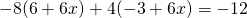Chapter 2: Linear Equations

# 2.3 Intermediate Linear Equations

When working with linear equations with parentheses, the first objective is to isolate the parentheses. Once isolated, the parentheses can be removed and then the variable solved.

Example 2.3.1

Solve for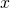in the equation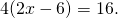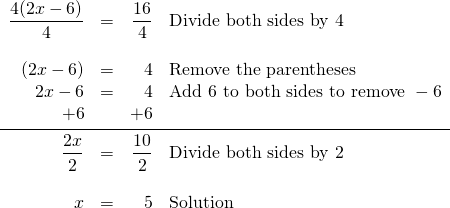Example 2.3.2

Solve forin the equation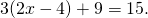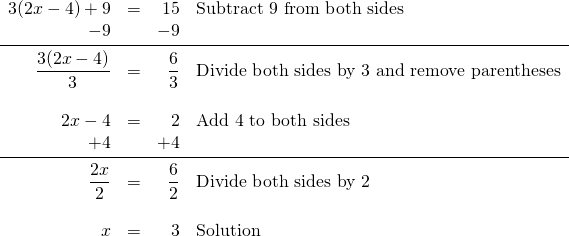For some problems, it is too difficult to isolate the parentheses. In these problems, it is necessary to multiply or divide throughout the parentheses by whatever coefficient is in front of it.

Example 2.3.3

Solve forin the equation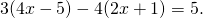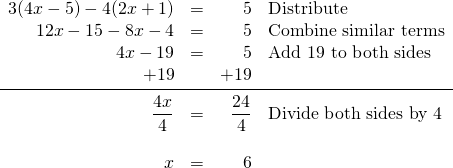# Questions

For questions 1 to 26, solve each linear equation.

1.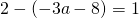2.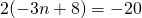3.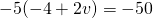4.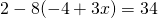5.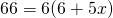6.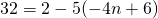7.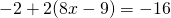8.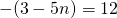9.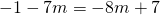10.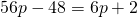11.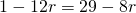12.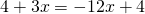13.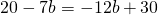14.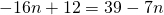15.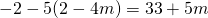16.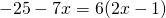17.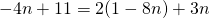18.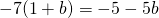19.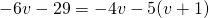20.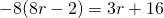21.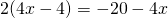22.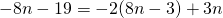23.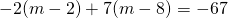24.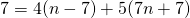25.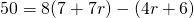26.